Muskan Gulati — Updated On October 11th, 2020

This article was published as a part of the Data Science Blogathon.

## INTRODUCTIONYay!! So you have successfully built your classification model. What should you do now?  How do you evaluate the performance of the model that is how good the model is in predicting the outcome. To answer these questions, let’s understand the metrics used in evaluating a classification model using a simple case study.

## LETS DEEP DIVE INTO CONCEPTS THROUGH CASE STUDY

In these COVID times, people are often traveling from one place to another. The airport can be a risk as passengers wait in queues, check-in for flights, visit food vendors, and use facilities such as bathrooms. Tracking COVID positive passengers at airports can help prevent the spread of the virus.Consider, we have a machine learning model classifying passengers as COVID positive and negative. When performing classification predictions, there are four types of outcomes that could occur:

True Positive (TP): When you predict an observation belongs to a class and it actually does belong to that class. In this case, a passenger who is classified as COVID positive and is actually positive.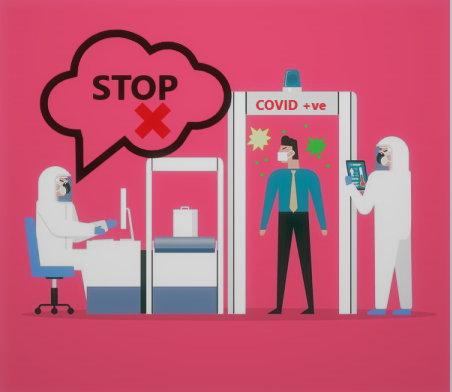Fig.1 – True Positive

True Negative (TN): When you predict an observation does not belong to a class and it actually does not belong to that class. In this case, a passenger who is classified as not COVID positive (negative) and is actually not COVID positive (negative).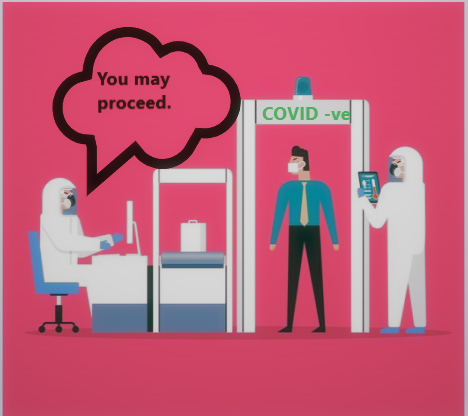Fig.2 – True Negative

False Positive (FP): When you predict an observation belongs to a class and it actually does not belong to that class. In this case, a passenger who is classified as COVID positive and is actually not COVID positive (negative).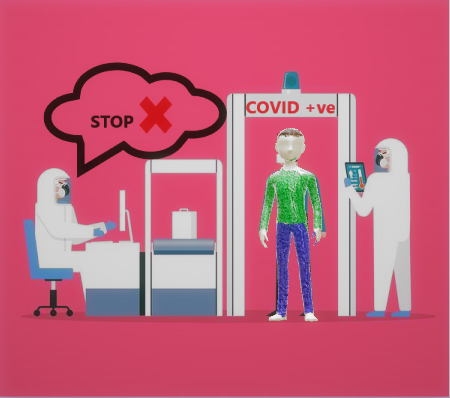Fig.3 – False Positive

False Negative(FN): When you predict an observation does not belong to a class and it actually does belong to that class. In this case, a passenger who is classified as not COVID positive (negative) and is actually COVID positive.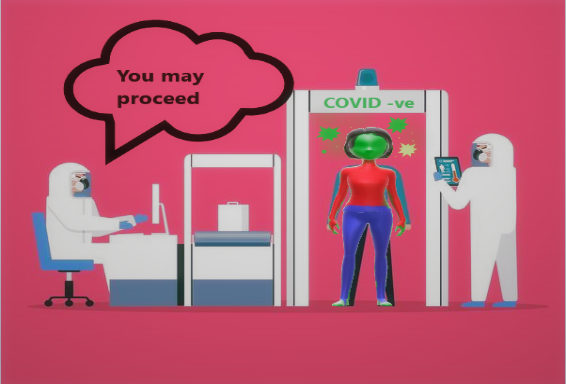Fig.4 – False Negative

## Confusion Matrix

For better visualization of the performance of a model, these four outcomes are plotted on a confusion matrix.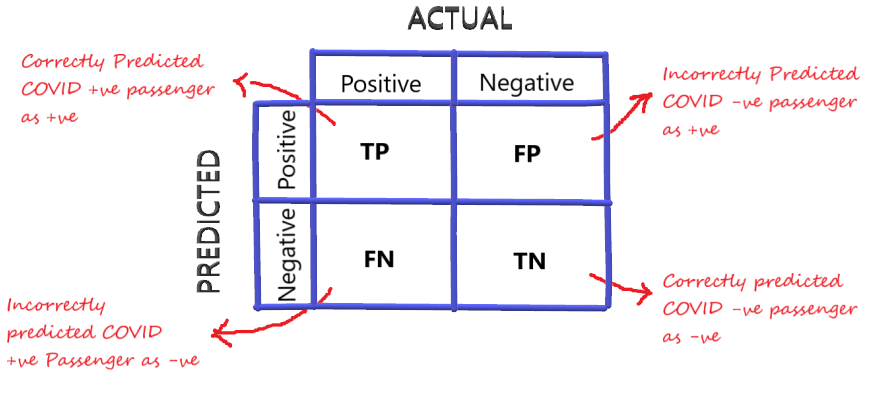Fig.5 Confusion Matrix

## Accuracy

Yes! You got that right, we want our model to focus on True positive and True Negative. Accuracy is one metric which gives the fraction of predictions our model got right. Formally, accuracy has the following definition:

Accuracy = Number of correct predictions / Total number of predictions.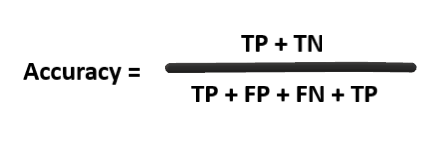Fig.-6

Now, let’s consider 50,000 passengers travel per day on an average. Out of which, 10 are actually COVID positive.

One of the easy ways to increase accuracy is to classify every passenger as COVID negative. So our confusion matrix looks like: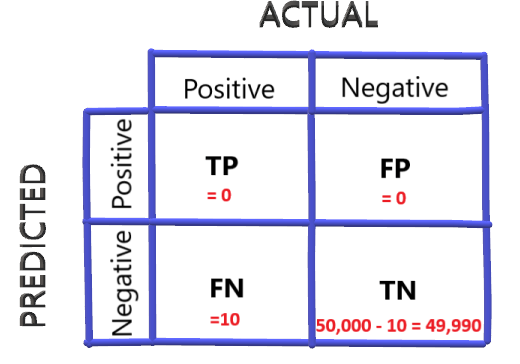Accuracy for this case will be:

Accuracy = 49,990/50,000 = 0.9998 or 99.98%Impressive!! Right? Well, does that really solve our purpose of classifying COVID positive passengers correctly?

For this particular example where we are trying to label passengers as COVID positive and negative with the hope of identifying the right ones, I can get 99.98% accuracy by simply labeling everyone as COVID negative. Obviously, this is a way more accuracy than we have ever seen in any model. But it doesn’t solve the purpose. The purpose here is to identify COVID positive passengers. Not labeling 10 of actually positive is a lot more expensive in this scenario. Accuracy in this context is a terrible measure because its easy to get extremely good accuracy but that’s not what we are interested in.

So in this context accuracy is not a good measure to evaluate a model. Let’s look at a very popular measure called Recall.

## Recall (Sensitivity or True positive rate)

Recall gives the fraction you correctly identified as positive out of all positives.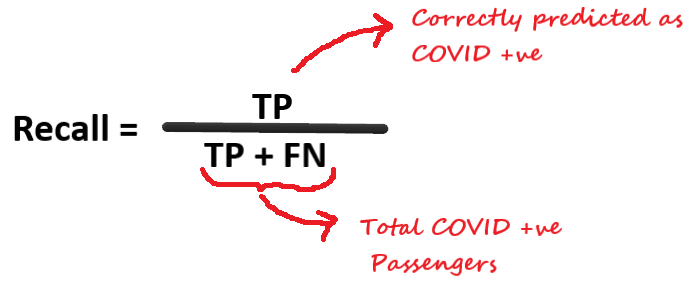Now, this is an important measure. Out of all positive passengers what fraction you identified correctly. Going back to our previous strategy of labeling every passenger as negative that will give recall of Zero.

Recall = 0/10 = 0

So, in this context, Recall is a good measure. It says that the terrible strategy of identifying every passenger as COVID negative leads to zero recall. And we want to maximize the recall.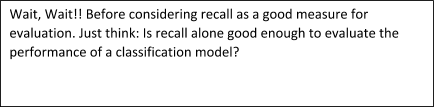To answer the above question, consider another scenario of labeling every passenger as COVID positive. Everybody walks into the airport and the model just labels them as positive. Labeling every passenger as positive is bad in terms of the amount of cost that needs to be spent in actually investigating each one before they board the flight.

The confusion matrix will look like: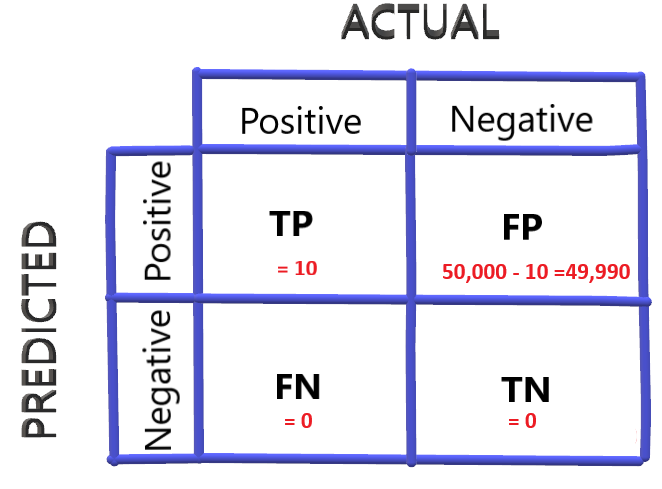Recall for this case would be:

Recall =  10/(10+0) = 1

That’s a huge problem. So concluding, it turns out that accuracy was a bad idea because labeling everyone as negative can increase the accuracy but hoping Recall will be a good measure in this context but then realized that labeling everyone as positive will increase recall as well.

So recall independently is not a good measure.

There is another measure called Precision

## Precision

Precision gives the fraction of correctly identified as positive out of all predicted as positives.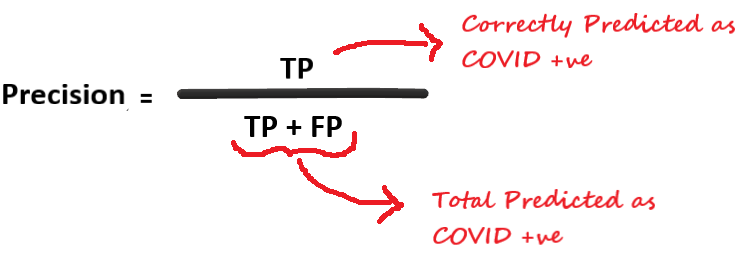Considering our second bad strategy of labeling every passenger as positive, the precision would be :

Precision =  10 / (10 + 49990) = 0.0002

While this bad strategy has a good recall value of 1 but it has a terrible precision value of 0.0002.

This clarifies that recall alone is not a good measure, we need to consider precision value.

Considering another Case (This will be the last one, I promise :P) of labeling the top passengers as COVID positive that is labeling passengers with the highest probability of having COVID. Let’s say we got only one such passenger. The confusion matrix in this case will be: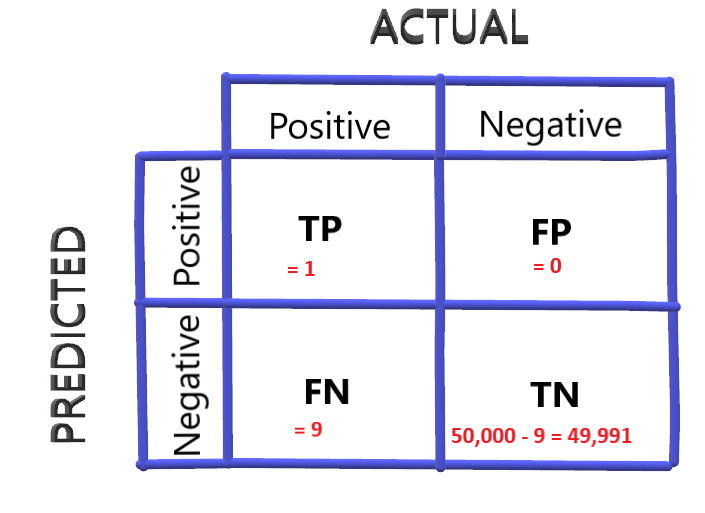Precision comes out to be: 1/ (1 + 0) = 1

Precision value is good in this case but let’s check for recall value once:

Recall = 1 / (1 + 9) = 0.1

Precision value is good in this context but recall value is low.

 Scenario Accuracy Recall Precision Classifying all the passengers as –ve High Low Low Classifying all the passengers as positive Low High Low Classifying passengers with max. probability as positive High Low Low

In some cases, we are pretty sure that we want to maximize either recall or precision at the cost of others. As in this case of labeling passengers, we really want to get the predictions right for COVID positive passengers because it is really expensive to not predict the passenger right as allowing COVID positive person to proceed will result in increasing the spread. So we are more interested in recall here.

Unfortunately, you can’t have it both ways: increasing precision reduces recall and vice versa. This is called precision/recall tradeoff.

Some Classification models output the probabilities between 0 and 1. In our case of classifying passengers as COVID positive and negative, where we want to avoid missing actual cases of positive.  In particular, if a passenger is actually positive but our model fails to identify it, that would be very bad because there is a high chance that the virus can be spread by allowing these passengers to board the flight. So, we can’t take the risk of labeling passengers as negative even with a small doubt of having COVID.

So we strategize in such a way that if the output probability is greater than 0.3, we label them as COVID positive.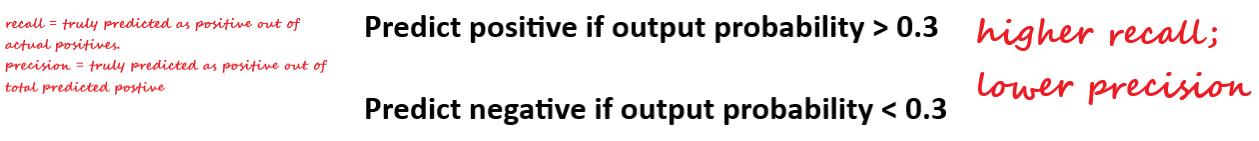This results in higher recall and lower precision.

Consider the opposite of this, where we want to classify the passenger as positive when we are sure that the passenger is positive. We set the probability threshold as 0.9 that is classifying passenger as positive when the probability is greater than or equal to 0.9 otherwise negative.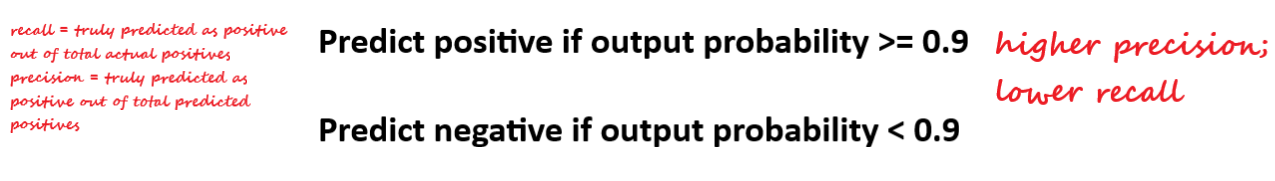So in general for most classifiers, there is going to be a trade-off between recall and precision as you vary the probability threshold.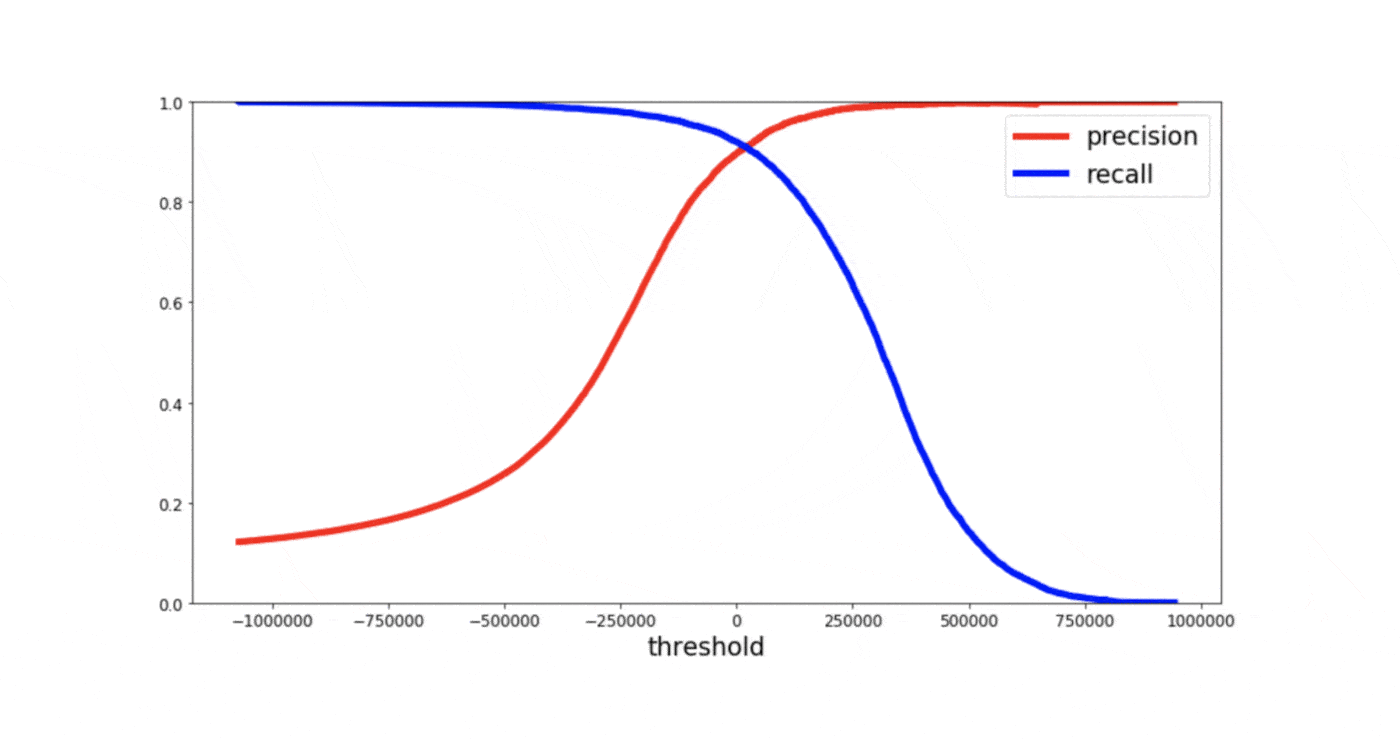If you need to compare different models with different precision-recall value, It is often convenient to combine precision and recall into a single metric. Correct!! We need a metric that considers both recall and precision to compute the performance.

## F1 Score

It is defined as the harmonic mean of the model’s precision and recall.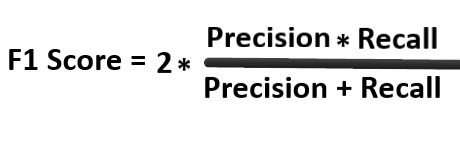You must be wondering why Harmonic mean and not simple average? You’re heading in the right direction.

We use Harmonic mean because it is not sensitive to extremely large values, unlike simple averages. Say, we have a model with a precision of 1, and recall of 0 gives a simple average as 0.5 and an F1 score of 0. If one of the parameters is low, the second one no longer matters in the F1 score. The F1 score favors classifiers that have similar precision and recall. Thus, the F1 score is a better measure to use if you are seeking a balance between Precision and Recall.

## ROC/AUC Curve

The receiver operator characteristic is another common tool used for evaluation. It plots out the sensitivity and specificity for every possible decision rule cutoff between 0 and 1 for a model. For classification problems with probability outputs, a threshold can convert probability outputs to classifications. we get the ability to control the confusion matrix a little bit. So by changing the threshold, some of the numbers can be changed in the confusion matrix. But the most important question here is, how to find the right threshold? Of course, we don’t want to look at the confusion matrix every time the threshold is changed, therefore here comes the use of the ROC curve.

For each possible threshold, the ROC curve plots the False positive rate versus the true positive rate.

False Positive Rate: Fraction of negative instances that are incorrectly classified as positive.

True Positive Rate: Fraction of positive instances that are correctly predicted as positive.

Now, think about having a low threshold. So amongst all the probabilities arranged in ascending order, everything below bad is considered as negative and everything above 0.1 is considered as positive. By choosing this, you’re being very liberal.

##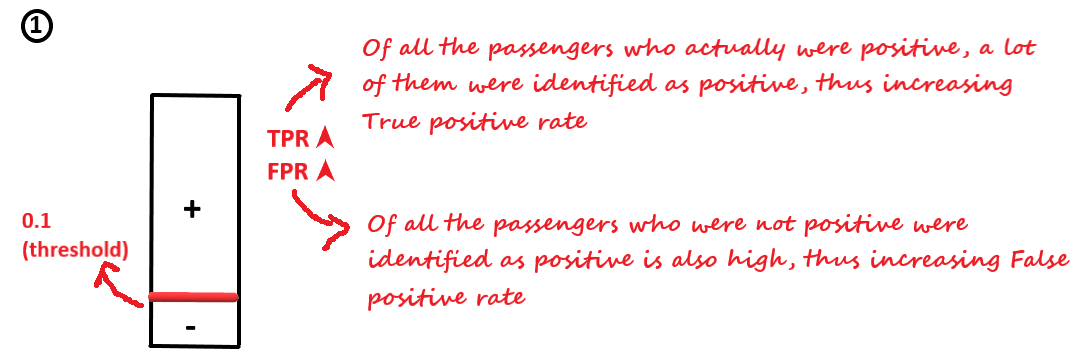But if you set your threshold as high, say 0.9.

##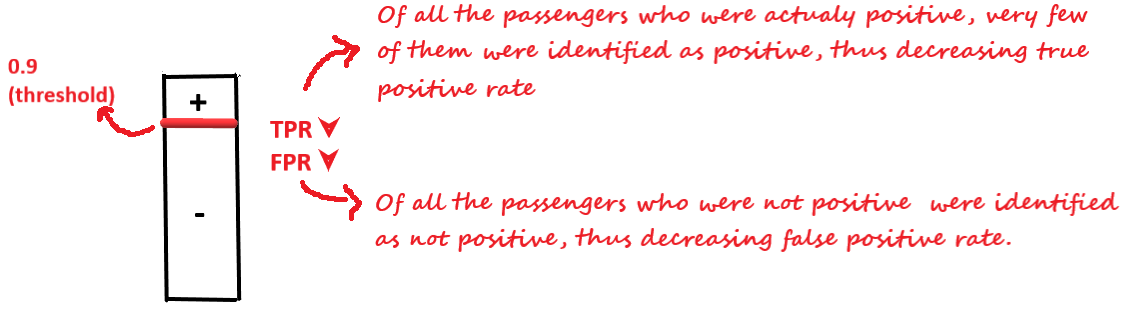Below is the ROC curve for the same model at different threshold values.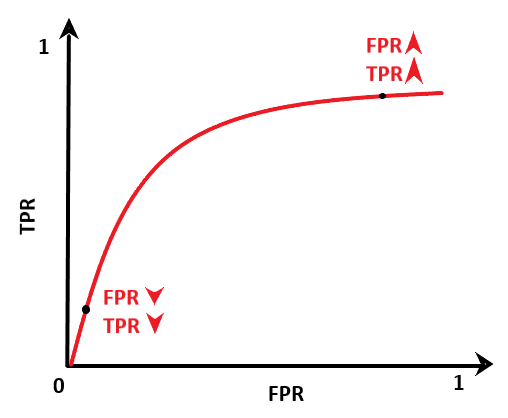From the above graph, it can be seen that the true positive rate increases at a higher rate but suddenly at a certain threshold, the TPR starts to taper off. For every increase in TPR, we have to pay a cost, the cost of an increase in FPR. At the initial stage, the TPR increase is higher than FPR

So, we can select the threshold for which the TPR is high and FPR is low.

Now, let’s see what different values about TPR and FPR tell us about the model.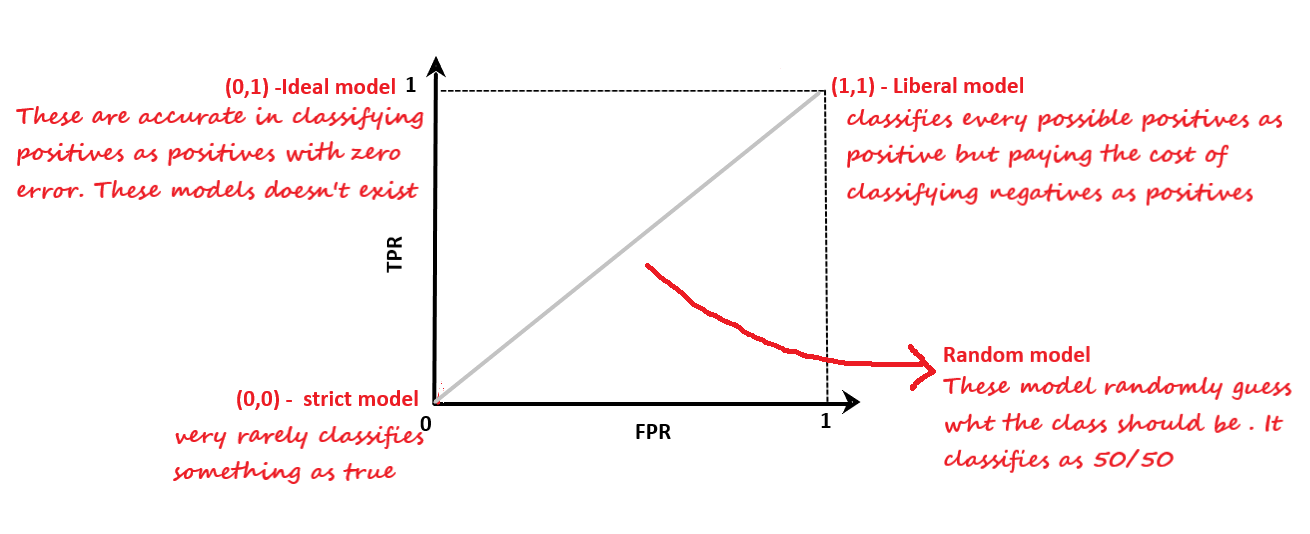For different models, we will have a different ROC curve. Now, how to compare different models? From the above plot, it is clear that the curve is in the upper triangle, the good the model is.  One way to compare classifiers is to measure the area under the curve for ROC.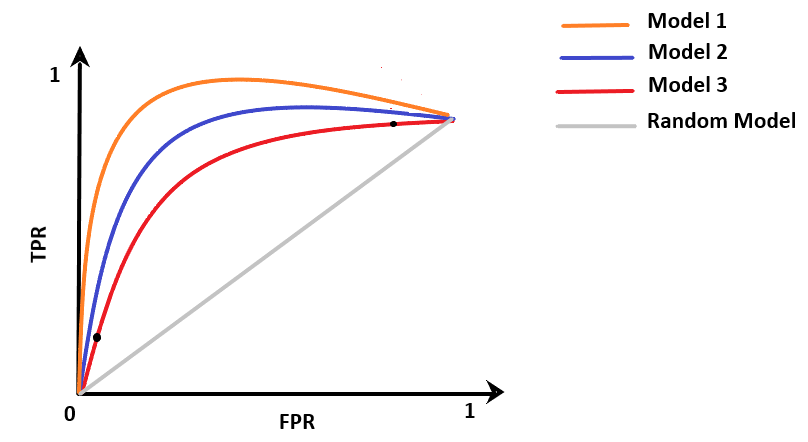AUC(Model 1) > AUC(Model 2) > AUC(Model 2)

Thus Model 1 is the best of all.

## Summary

We have learned different metrics used to evaluate the classification models. When to use which metrics depends primarily on the nature of your problem. So get back to your model now, question yourself what is the main purpose you are trying to solve, select the right metrics, and evaluate your model.### Our Top Authors

•